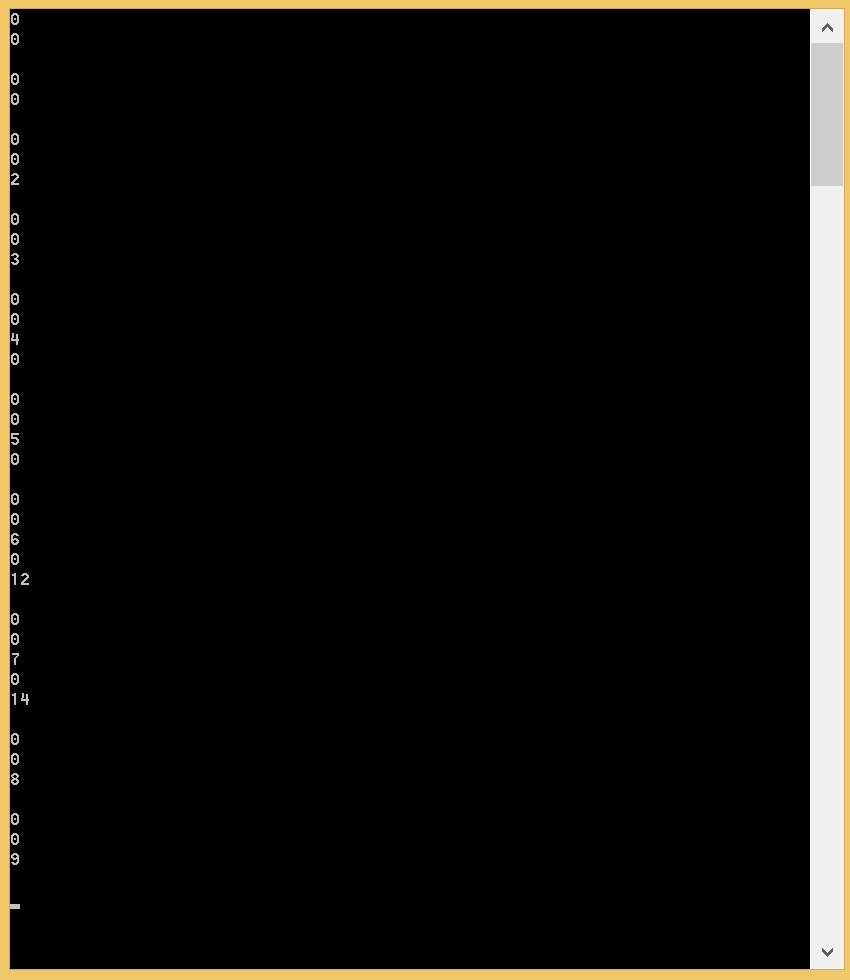# Gergely Kalapos

## Marshalling Jagged Arrays from CLR to Native

Posted on August 02, 2014

This week I was working on some stuff where I calculated some values in C# and I wanted to send it to a native C++ layer to use the values there. The data was originally stored in a Dictionary<int, List>. As soon as I realised that I want to work with this in C++ it was clear that the Dictinary and the List classes are not the best, so instead of the C# collections I used a C# jagged array and I hoped that the framework can marshal it automatically. Well I was naive… If you try to marshal a jagged array you get an exception.

In this post I would like to show how I solved this problem.

To show this I created two Visual Studio projects. One native C++ project to create a DLL and a C# console application which uses the DLL via PInvoke.

First let’s see the C# code.

There is one method to create the jagged array:

``````
static long[][] initArray(int numberOfSubArrays, int[] sizeOfSubArray)
{
long[][] retVal = new long[numberOfSubArrays][];
for (int i = 0; i < numberOfSubArrays; i++)       {
retVal[i] = new long[sizeOfSubArray[i]];

for (int j = 0; j < sizeOfSubArray[i]; j++)
{
retVal[i][j] = (i * j);
}
}
return retVal;}

``````

Nothing special here. This is just a pure C# function which creates the jagged array. The number of subarrays is the first parameter and the size of every subarray is stored in the second parameter. Please note that I do not use any range check here because I want to keep the example simple.

Now let’s see the method which uses the array:

``````

static void Main(string[] args)
{
int nofSubArrays = 10;
int[] sizeOfSubArray = { 2, 2, 3, 3, 4, 4, 5, 5, 3, 3 };

long[][] arrayToMarshal = initArray(nofSubArrays, sizeOfSubArray);

IntPtr[] subarrays = new IntPtr[nofSubArrays];

for (int i = 0; i < nofSubArrays; i++)
{
subarrays[i] = Marshal.AllocHGlobal(sizeOfSubArray[i] *                sizeof(ulong));
Marshal.Copy(arrayToMarshal[i], 0, subarrays[i],                sizeOfSubArray[i]);
}

ProcessJaggedArray(nofSubArrays, sizeOfSubArray, subarrays);

for (int i = 0; i < nofSubArrays; i++)
{
Marshal.FreeHGlobal(subarrays[i]);
}

}

``````

First we create the array which contains 10 subarray. The size of each subarray is in the sizeOfSubArray. Our goal is to Marshal this jagged array into the native layer via PInvoke. As I mentioned there is no way to Marshal a long[][] directly.

My idea is that I send an IntPtr array to C++ where every pointer points to the first item of the subarray. In order to keep track of the sizes of the subarrays I also send an additional array which is in our case the sizeOfSubArray.

So the IntPre[] subarrays is the pointer array.

Now let’s examine the for loop.

``subarrays[i] = Marshal.AllocHGlobal(sizeOfSubArray[i] * sizeof(ulong));``

The Marshal.AllocHGlobal method allocates memory on the unmanaged heap and returns a pointer which points to the allocated memory (and this is in C#! Isn't that cool?!). So in this line we allocate memory for every i. subarray.

The second line

``````Marshal.Copy(arrayToMarshal[i], 0, subarrays[i], sizeOfSubArray[i]);
``````

copies a C# array into an unmanaged memory space. The first parameter of the copy method is the array itself which we want to copy the second is the index of the first element which we want to copy the third one is the destination address (a pointer) and the fourth is the number of items we want to copy.

So basically the first line allocates memory on the unmanaged heap the second one writes the values to the unmanaged address space.

The next line is the PInvoke call to the native ProcessJaggedArray method and after that the allocated memory is freed so the application does not leak memory.

The declaration the native function in C# is this:

``````
[DllImport(@"../../../Debug/NativeDll.dll", CharSet = CharSet.Auto,  ExactSpelling = true, CallingConvention = CallingConvention.Cdecl)]
static extern int ProcessJaggedArray(int NumberOfSubArrays,  int[] sizeOfSubarrays, IntPtr[] subArrays);

``````

Now let’s see how is this implemented in C++.

First in the header file I defined the function:

``````extern "C" __declspec(dllexport)  void ProcessJaggedArray(int NumberOfSubArrays, int sizeOfSubarrays[], long* marshaledArray[]);
``````

As you can see I use c calling convention (note that this is also defined in the C# DllImport attribute). The third parameter is the array itself. Instead of long* marshaledArray[] I also could write long**, but I wanted to emphasise that I treat this long** as a pointer of long arrays (Yes..this is a bit of a philosophical question, since this is also a long**….).

And now the implementation:

``````__declspec(dllexport)  void ProcessJaggedArray(int NumberOfSubArrays, int sizeOfSubarrays[], long* marshaledArray[])
{
for (size_t i = 0; i < NumberOfSubArrays; i++)
{
for (size_t j = 0; j < sizeOfSubarrays[i]; j++)
{
std::cout << marshaledArray[i][j] << std::endl;
}

std::cout << std::endl;
}
}

``````

Instead of really processing the values they are just printed out to the console in order to keep the example simple. The point is that we can treat the IntPtr[] sent from C# as an array of a pointers where each pointer points to an array of longs.

Finally the output of the running program:The Visual Studio solution can be found here.

;